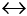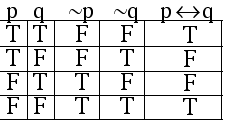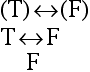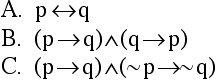UNIT 1 MODULE 6

THE BICONDITIONAL STATEMENT

Compare the following two statements:

1. If you are a slacker, then you will get fired.

2. If you are a slacker, then you will get fired, and if you aren't a slacker, then you won't get fired.

Notice that the second statement conveys some crucial information that the first statement omits; namely, first statement doesn't stipulate what must happen if you aren't a slacker, whereas the second statment does provide such information.

The second statement is an example of a biconditional statement. Biconditional statements are usually phrased with the connective "if and only if." A more concise way to formulate statement 2 above is as follows:

2. You will get fired if and only if you are a slacker.

The "if and only if" connective is represented by this symbol:TRUTH BEHAVIOR of the BICONDITIONAL STATEMENT

In general, a statement of the form "A if and only if B" will be TRUE when A has the same truth value as B, and FALSE when A and B have differing truth values.

EXAMPLE 1.6.1

Determine the truth value of each statement.

A. Atlanta isn't in Georgia if and only if 1 + 1 = 2.

B. All cats are reptiles if and only if all dogs are fish.

EXAMPLE 1.6.1 SOLUTIONS

A. Atlanta isn't in Georgia if and only if 1 + 1 = 2

This statement has the form "p if and only if q," where p (Atlanta isn't in Georgia) is a false statement while q ("1 + 1 = 2") is a true statement. Since the two components of this biconditional statement have opposite truth values, the statement is FALSE.

B. All cats are reptiles if and only if all dogs are fish.

This statement has the form "p if and only if q," where p ("All cats are reptiles") is a false statement while q ("All dogs are fish") is also a false statement. Since the two components of this biconditional statement have the same truth values, the statement is TRUE.

THE TRUTH TABLE FOR THE BICONDITIONAL STATEMENT

Bearing in mind the truth bevavior described above, the "if and only if" statement must have the following truth table:Notice that the statement is true in the first and fourth rows, where the truth value of p is the same as the truth value of q. On the other hand, the statement is false in the second and third rows, where p and q have opposite truth values.

EXAMPLE 1.6.2

Suppose q represents a true statement while p and r represent false statements.
Find the truth value ofEXAMPLE 1.6.2 SOLUTION

We will substitute the given values for their respective variables in the expressionLetting q be "T" and letting p and r be "F" we have:Now we simplify the negations within the left-hand parentheses:Next we simplify the expressions within each set of parentheses. The disjunction inside of the left-hand parentheses is TRUE, while the conditional within the right-hand parentheses is FALSE.Because the statement simplified to a biconditional in which the two components have opposite truth values, the statement is FALSE.

WWW note:
For more practice involving symbolic statements, see The Logicizer

EXAMPLE 1.6.3 Use a truth table to verify that the following statements are all equivalent:WWW note:
For more practice involving truth tables, see The Truth Tabler